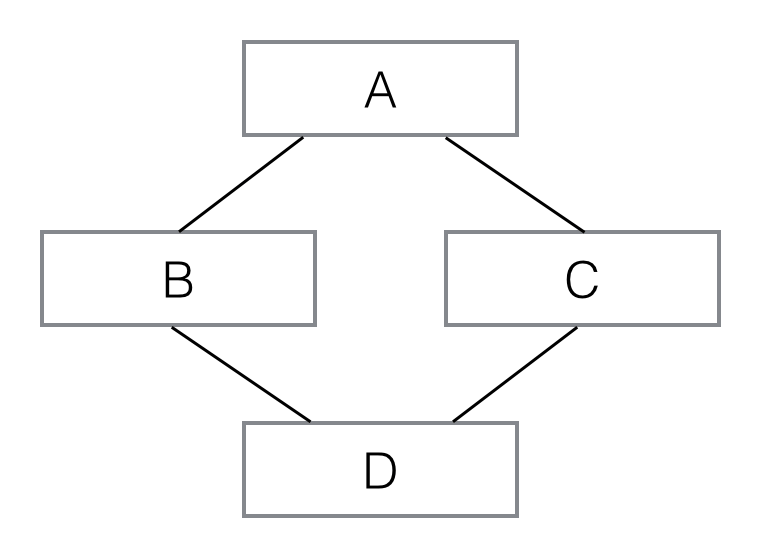# Python 中的类与继承

## 类的定义以及实例的建立

Python中，类通过 class 关键字定义。

```class Person(object):
pass```

Python 的编程习惯，类名以大写字母开头，紧接着是(object)，表示该类是从哪个类继承下来的。

```zhangsan=Person()
lisi=Person()```

## 实例的属性及方法

Python是动态语言，对每一个实例，都可以直接给他们的属性赋值，即使类中并无定义，例如，给xiaoming这个实例加上name、gender和birth属性：

```xiaoming = Person()
xiaoming.name = 'Xiao Ming'
xiaoming.gender = 'Male'
xiaoming.birth = '1990-1-1'```

### __init__()

```class Person(object):
def __init__(self, name, gender, birth):
self.name = name
self.gender = gender
self.birth = birth```

__init__() 方法的第一个参数必须是 self（也可以用别的名字，但建议使用习惯用法），后续参数则

```xiaoming = Person('Xiao Ming', 'Male', '1991-1-1')
xiaohong = Person('Xiao Hong', 'Female', '1992-2-2')```

### 属性的访问限制（私有属性）

Python对属性权限的控制是通过属性名来实现的，如果一个属性由双下划线开头(__)，该属性就无

```class Person(object):
def __init__(self, name):
self.name = name
self._title = 'Mr'
self.__job = 'Student'

p1=Person("zhangsan")

print(p1.name,'\n')
print(p1._title,'\n')
try:
print(p1.__job)
except:
print("Error")```

```zhangsan

Mr

Error```

### 实例的方法

```class Person(object):

def __init__(self, name):
self.__name = name

def get_name(self):
return self.__name

p2=Person("Bob")
print(p2.get_name())```

`Bob`

get_name(self) 就是一个实例方法，它的第一个参数是self。__init__(self, name)其实也可看做是一个特殊的实例方法。

### python中实例方法也是属性

```import types
def Pn_get_name(self):
return str("This person's name is :"+self.name)

class Person(object):
def __init__(self, name):
self.name = name

p1=Person("Alice")
p2=Person("Bob")

p1.get_name=types.MethodType(Pn_get_name,p1)

print(p1.get_name(),'\n')
print(p2.get_name())```

```This person's name is :Alice

Traceback (most recent call last):
File "D:/pythonwork/practise/test.py", line 15, in <module>
print(p2.get_name())
AttributeError: 'Person' object has no attribute 'get_name'```

## 类的属性及方法

```class Person(object):
def __init__(self, name):
self.name = name```

```class Person(object):
def __init__(self, name):
self.name = name

p1=Person("zhangsan")

```Earth
Earth```

```Person.address = 'China'

```China
China```

```p1.address="China"

```Earth
China```

### 类的方法

```class Person(object):
count = 0
@classmethod
def how_many(cls):
return cls.count

def __init__(self, name):
self.name = name
Person.count = Person.count + 1

print (Person.how_many())
p1 = Person('Bob')
print (Person.how_many())```

```0
1```

## 类的继承

```class Person(object):
def __init__(self, name, gender):
self.name = name
self.gender = gender

class Student(Person):
def __init__(self, name, gender, score):
super(Student, self).__init__(name, gender)
self.score = score```

### 初始化父类的多种写法

```class AAA(object):
def __init__(self, name):
self.name = name

def get_name(self):
print(self.name)
return self.name

class BBB(AAA):
def __init__(self, age):
self.age = age
# 方式1
super().__init__(name='BBB')
# 方式2
super(BBB, self).__init__(name='BBBC')
# 方式3
AAA.__init__(self, name='BBBD')

def get_age(self):
print(self.age)
return self.age```

```class AAA():
def __init__(self, name):
self.name = name

def get_name(self):
print(self.name)
return self.name

class BBB():
def __init__(self, name2):
self.name2 = name2

def get_name2(self):
print(self.name2)
return self.name2

class CCC(AAA, BBB):
def __init__(self, age):
self.age = age
AAA.__init__(self, name='CCC')
BBB.__init__(self, name2='CCC2')

def get_age(self):
print(self.age)
return self.age

TEST = CCC(2)
TEST.get_name()
TEST.get_name2()```

### python中的多态

Teacher ，并都写了一个 同名的whoAmI() 方法，当我们调用实例的whoAmI() 方法时，总是先查找

```class Person(object):
pass
def Pstr(self):
print("This is Person class method")

class Student(Person):
def __init__(self, name):
self.name = name
def Pstr(self):
print("This is Student class method")

class Teacher(Person):
def __init__(self, name):
self.name = name

p1=Student("Alice")
p2=Teacher("Tom")

p1.Pstr()
p2.Pstr()```

```This is Student class method
This is Person class method```

### python中的多重继承

```class A(object):
def __init__(self, a):
print 'init A...'
self.a = a

class B(A):
def __init__(self, a):
super(B, self).__init__(a)
print 'init B...'

class C(A):
def __init__(self, a):
super(C, self).__init__(a)
print 'init C...'

class D(B, C):
def __init__(self, a):
super(D, self).__init__(a)
print 'init D...'```__init__()方法时，A 虽然被继承了两次，但__init__()只调用一次：

运行测试代码：

`d=D('d')`

```init A...
init C...
init B...
init D...```

## 类的定制

### python中 __slots__

```class Student(object):
__slots__ = ('name', 'gender', 'score')
def __init__(self, name, gender, score):
self.name = name
self.gender = gender
self.score = score```

```>>> s = Student('Bob', 'male', 59)
>>> s.name = 'Tim' # OK
>>> s.score = 99 # OK
Traceback (most recent call last):
...
AttributeError: 'Student' object has no attribute 'grade'```

__slots__的目的是限制当前类所能拥有的属性，如果不需要添加任意动态的属性，使用__slots__也能节省内存。

### python中 __str__和__repr__对于print的改变

```class Student(object):
def __init__(self, name):
self.name = name

p1=Student("Alice")

print(p1)```

`<__main__.Student object at 0x000002672671EF98>`

```class Student(object):
def __init__(self, name):
self.name = name
def __str__(self):
return 'Student: %s' % self.name

p1=Student("Alice")

print(p1)```

`Student: Alice`

```>>>p1
<Student object at 0x0000023C8F331A58>```

`__repr__ = __str__`

```>>>p1
Student: Alice```

### python中 __len__对于len()的改变

```class Students(object):
def __init__(self, *args):
self.names = args
def __len__(self):
return len(self.names)

ss = Students('Bob', 'Alice', 'Tim')
print (len(ss))```

`3 `

### 类的实例的数学运算

__sub__对符号 -进行改变

__mul__对符号 * 进行改变

__div__对符号 / 进行改变

```class Rational(object):
def __init__(self, p, q):
self.p = p
self.q = q```

```class Rational(object):
def __init__(self, p, q):
self.p = p
self.q = q
return Rational(self.p * r.q + self.q * r.p, self.q * r.q)
def __str__(self):
return '%s/%s' % (self.p, self.q)
__repr__ = __str__

r1 = Rational(1, 3)
r2 = Rational(1, 2)
print(r1+r2)```

`5/6`

### 类的实例的类型转换

```class Rational(object):
def __init__(self, p, q):
self.p = p
self.q = q
def __int__(self):
return self.p//self.q   #python3中//是整数结果```

```>>> print int(Rational(7, 2))
3```

### python中 @property

```class Student(object):
def __init__(self, name, score):
self.name = name
self.score = score```

```s = Student('Bob', 59)
s.score = 1000```

```class Student(object):
def __init__(self, name, score):
self.name = name
self.__score = score
def get_score(self):
return self.__score
def set_score(self, score):
if score < 0 or score > 100:
raise ValueError('invalid score')
self.__score = score```

```class Student(object):
def __init__(self, name, score):
self.name = name
self.__score = score
@property
def score(self):
return self.__score
@score.setter
def score(self, score):
if score < 0 or score > 100:
raise ValueError('invalid score')
self.__score = score```

```s = Student('Bob', 59)
s.score = 60
print (s.score)
try:
s.score = 1000
except:
print("Error")```

```60
Error```

### python中 __call__

```>>> f = abs
>>> f.__name__
'abs'
>>> f(-123)
123```

```class Person(object):
def __init__(self, name, gender):
self.name = name
self.gender = gender

def __call__(self, friend):
print ('My name is %s...' % self.name)
print ('My friend is %s...' % friend)```
```>>> p = Person('Bob', 'male')
>>> p('Tim')
My name is Bob...
My friend is Tim...```